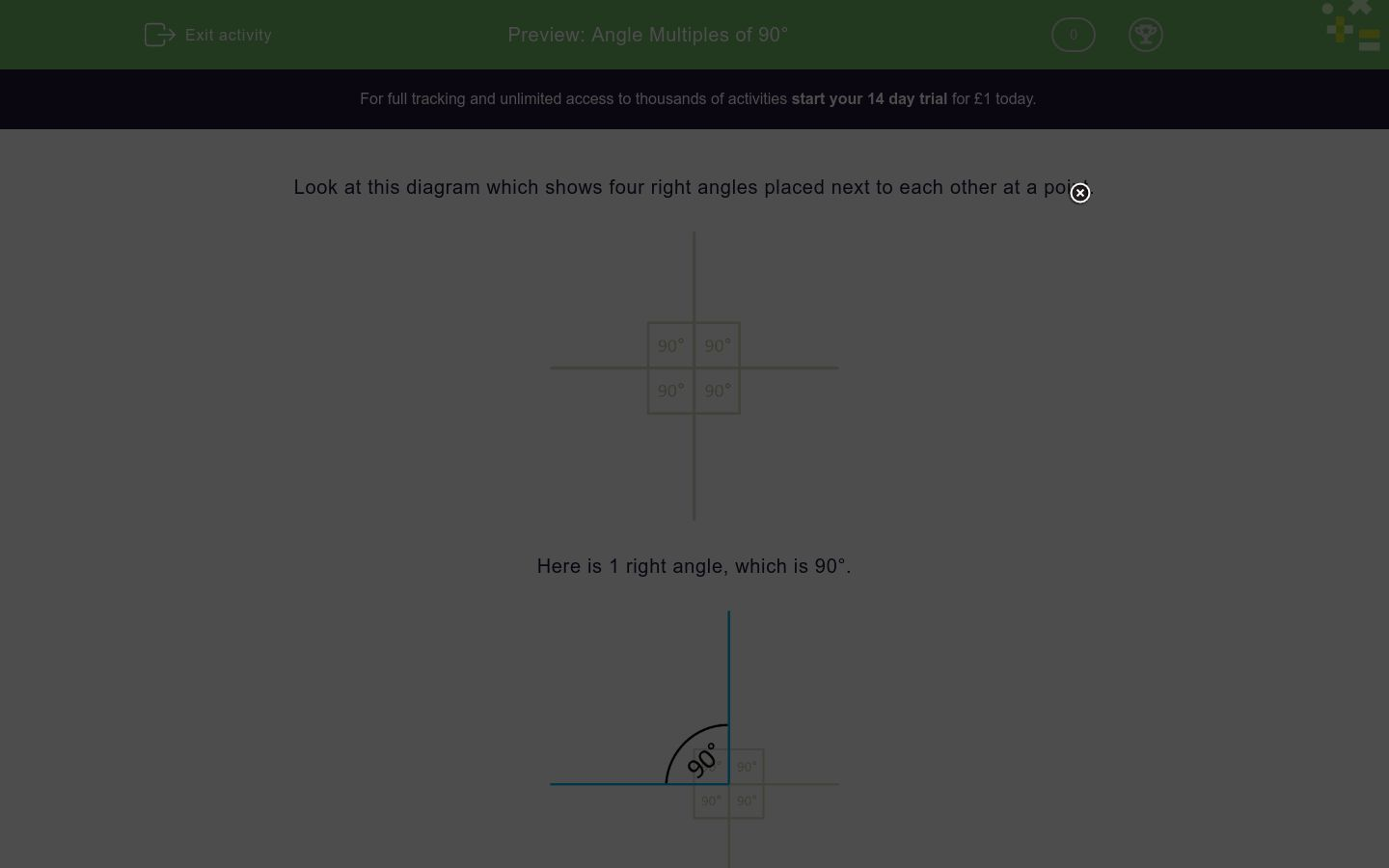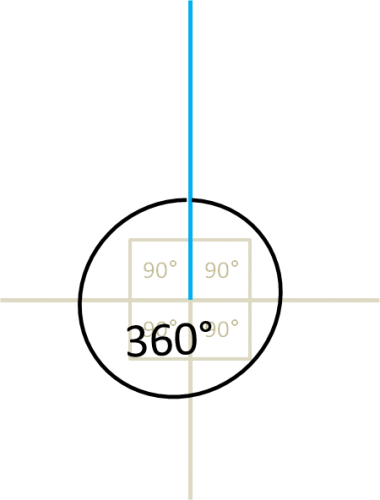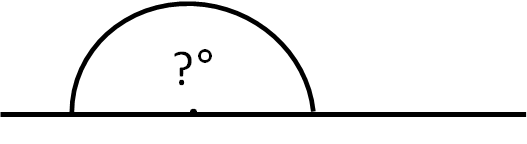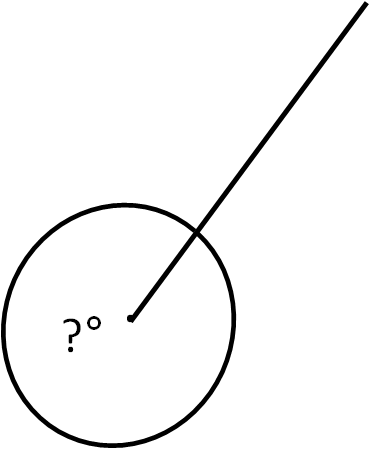# Angle Multiples of 90°

In this worksheet, students must identify angles which are multiples of 90° by counting right angles.Key stage:  KS 2

Curriculum topic:   Geometry: Properties of Shapes

Curriculum subtopic:   Identify Angles at a Point

Difficulty level:### QUESTION 1 of 10

Look at this diagram which shows four right angles placed next to each other at a point.Here is 1 right angle, which is 90°.Here are 2 right angles put together, giving an angle of 180°.

2 × 90° = 180°Here are 3 right angles put together, giving an angle of 270°.

3 × 90° = 270°Here are 4 right angles put together, giving an angle of 360°.

4 × 90° = 360°Want to understand this further and learn how this links to other topics in maths?

Why not watch this video?

Look at the diagram.

Select the correct value for the angle shown by the question mark.90°

180°

270°

360°

Look at the diagram.

Select the correct value for the angle shown by the question mark.90°

180°

270°

360°

Look at the diagram.

Select the correct value for the angle shown by the question mark.90°

180°

270°

360°

Look at the diagram.

Select the correct value for the angle shown by the question mark.90°

180°

270°

360°

Look at the diagram.

Select the correct value for the angle shown by the question mark.90°

180°

270°

360°

Look at the diagram.

Select the correct value for the angle shown by the question mark.90°

180°

270°

360°

Look at the diagram.

Select the correct value for the angle shown by the question mark.90°

180°

270°

360°

Look at the diagram.

Select the correct value for the angle shown by the question mark.

The angle goes right round as shown.90°

180°

270°

360°

Look at the diagram.

Select the correct value for the angle shown by the question mark.90°

180°

270°

360°

Look at the diagram.

Select the correct value for the angle shown by the question mark.90°

180°

270°

360°

• Question 1

Look at the diagram.

Select the correct value for the angle shown by the question mark.90°
EDDIE SAYS
One right angle.
• Question 2

Look at the diagram.

Select the correct value for the angle shown by the question mark.180°
EDDIE SAYS
Two right angles.
• Question 3

Look at the diagram.

Select the correct value for the angle shown by the question mark.360°
EDDIE SAYS
Four right angles.
• Question 4

Look at the diagram.

Select the correct value for the angle shown by the question mark.180°
EDDIE SAYS
Two right angles.
• Question 5

Look at the diagram.

Select the correct value for the angle shown by the question mark.270°
EDDIE SAYS
Three right angles.
• Question 6

Look at the diagram.

Select the correct value for the angle shown by the question mark.90°
EDDIE SAYS
One right angle.
• Question 7

Look at the diagram.

Select the correct value for the angle shown by the question mark.360°
EDDIE SAYS
Four right angles.
• Question 8

Look at the diagram.

Select the correct value for the angle shown by the question mark.

The angle goes right round as shown.270°
EDDIE SAYS
Three right angles.
• Question 9

Look at the diagram.

Select the correct value for the angle shown by the question mark.180°
EDDIE SAYS
Two right angles.
• Question 10

Look at the diagram.

Select the correct value for the angle shown by the question mark.270°
EDDIE SAYS
Three right angles.
---- OR ----